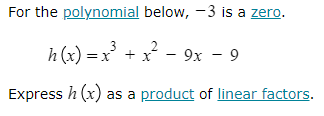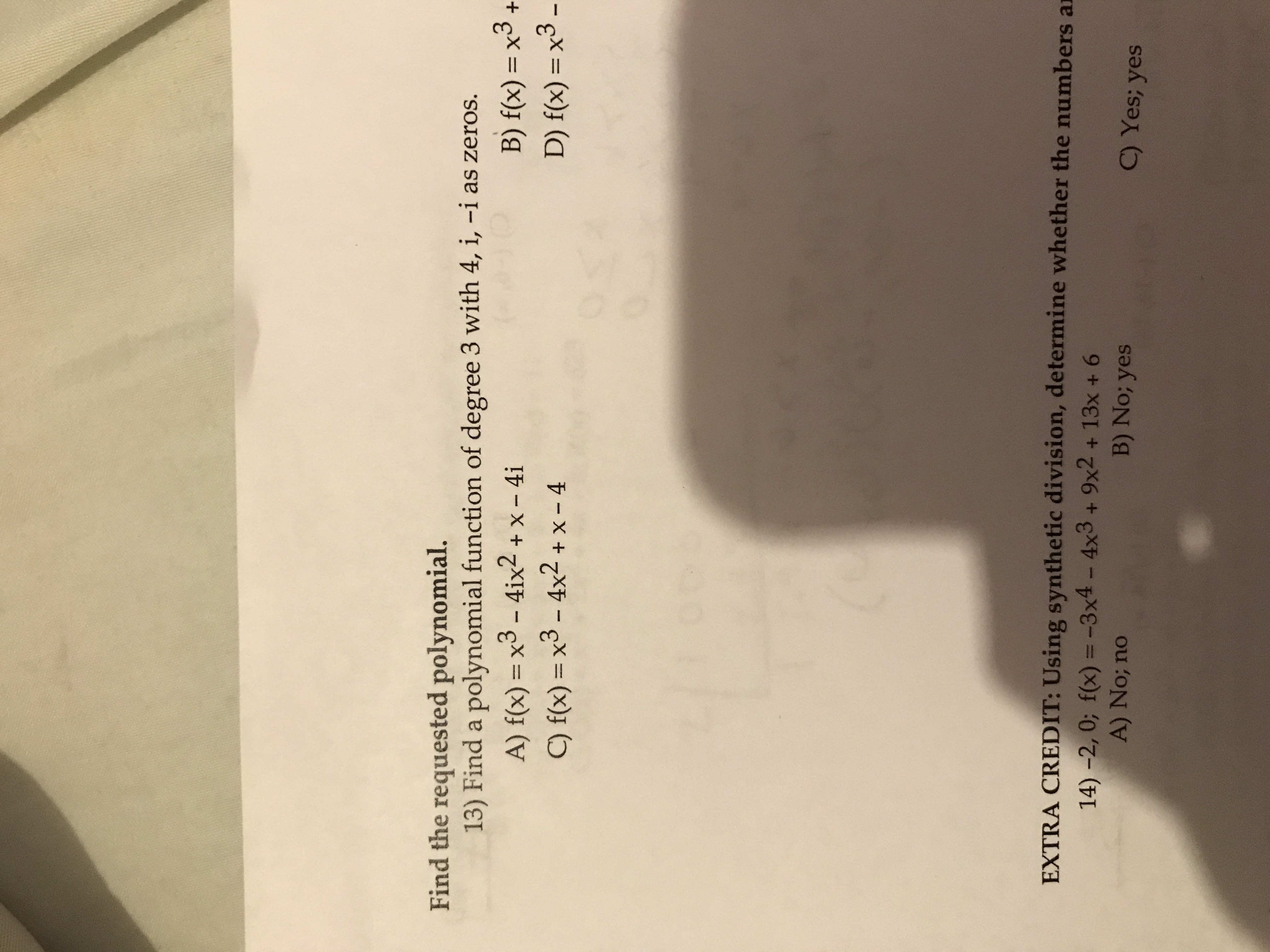Recent questions in Transformations of functions
College algebraOpen questionNatalia Del Vecchio2022-11-30

## Given that 1${\mathrm{log}}_{a}\left(3\right)\approx 0.61$  and l${\mathrm{log}}_{a}\left(5\right)\approx 0.9$ , evaluate each of the following. Hint: use the properties of logarithms to rewrite the given logarithm in terms of the the logarithms of 3 and 5.

College algebraOpen questionMelodie Gutknecht2022-11-02

## Find the formula for an exponential function that passes through (0,6) and (2,750)

College algebraOpen questionZakiya Noor2022-10-13

## A polynomial of a degree 5 had rational coefficients and the zeros  $\frac{4}{3},8i$, and $3-5\sqrt{2}$What are the missing zeros?elfmo20 2022-08-14

##Stephanie Wilkerson2022-08-09

## Perform the indicated operations and indicate the degree of the resulting polynomial.(-8x^5+6x^2-9x+11)+(19x^5+3x^2-3x-14)betterthennewzv 2022-08-08

## Given f(x) = absoulte value of X and g(x)= x-2. Find $\left(f+g\right)\left(x\right),\left(f-g\right)\left(x\right),\left(fg\right)\left(x\right),\left(f/g\right)\left(x\right)$ and $\left(f\circ g\right)\left(x\right)$Ismael Molina 2022-07-16

## Prove the identity$\mathrm{csc}x-\mathrm{cot}x\cdot \mathrm{cos}x=\mathrm{sin}x$

College algebraOpen questionAmanda Davila2022-06-03

## x=-3Fleurette1932 2022-05-15

##College algebraOpen question

## Write an equation for the graphed function by using transformations of the graphs of one of the toolkit functions. y =jamesvivian911 2022-02-08

## The function g is related to one of the parent functions g(x) = x^2 + 6 The parent function f is: f(x)= x^2 Use function notation to write g in terms of f.percibaa8 2021-12-20

## Suppose $f\left(x,y\right)=\left(x-y\right)\left(16-xy\right)$. Answer the following 1. Find the local maxima of $f$. 2. Find the local minima of $f$. 3. Find the saddle points of $f$.nicekikah 2021-09-30

## g is related to one of the parent functions. Describe the sequence of transformations from f to g. $g\left(x\right)=2-\left(x+5{\right)}^{2}$facas9 2021-09-30

## Given that f(x) = 3x - 7 and that (f + g)(x) = 7x + 3, find g(x).Rui Baldwin 2021-09-29

## use long division to determine if 2x-1 is a factor of $p\left(x\right)=6{x}^{3}+7{x}^{2}-x-2$jernplate8 2021-09-28

## Graph f and g in the same rectangular coordinate system. Use transformations of the graph off to obtain the graph of g. Graph and give equations of all asymptotes. Use the graphs to determine each function’s domain and range. $f\left(x\right)=\mathrm{ln}x\phantom{\rule{1em}{0ex}}\text{and}\phantom{\rule{1em}{0ex}}g⟨x\right)=-\mathrm{ln}\left(2x\right)$smileycellist2 2021-09-24

## Show that f is inverse of g and vice-versa, if f(x)=x-6 and g(x)=x+6Dolly Robinson 2021-09-23
## Find domain of fog, if 1. $f\left(x\right)=x+5;g\left(x\right)=\frac{7}{x+7}$ 2. $f\left(x\right)=\sqrt{x};g\left(x\right)=6x+18$banganX 2021-09-22